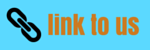# Class 9 Science test full course test (unsolved)

SA assignments

Question 1
1. What are canal rays?
2.  If one atom contains one electron and one proton, will it carry any charge or not?
3. What do you think would be the observation if the alpha-particles scattering experiment is carried out using a foil of a metal other than gold?
Question 2
1. Helium has an atomic mass of u and two protons in in its nucleus. How many neutrons does it have?
2. If K and L shells of an atom are full, then what would be the total number of electrons in the atom?
3. Write the electronic configuration of any one pair of isotopes.
Question 3
1. If Z=3 what would be the valency of the element? Also name the element.
2. Atoms of most elements are not able to exist independently.’ Name two elements which exist as independent atoms.
3. Name one element which forms diatomic and tera atomic molecules?
4. The chemical formula of oxide of an element ‘X’ is ‘X2O5’. Write the chemical formula of its chloride.
5. An element ‘X’ has mass number 4 and atomic number 2. Find the valency of ‘X’.

Question 4
1. Hydrogen and oxygen combine in the ratio of 1:8 by mass to form water. What mass of oxygen gas would be required to react completely with 3 gm of hydrogen gas?
2. Which postulate of Dalton’s theory can explain the law of definite proportions? Aluminium chloride and Sodium sulphide.

Question 5
1. Write down the name of compounds represented by Al2(SO4) and KNO3.
2. How many atoms are present in a PO4-3 .
3. Calculate the formula unit masses of ZnO and Na2O.
Question 6
1. Calculate the number of particles in 46 gm of Na atom.
2. Convert 20 gm of water into mole.
3. Give the name of elements present in quick lime and baking powder.
4. What is the primary characteristic on which the first division of organisms was made?
Question 7
1. On what basis are plants and animals put into different categories.
2. Which gas is the major component of the atmosphere of Venus and Mars?
3. Name the winds which bring rain in India.
4. Large scale deforestation should be stopped. List two reasons to justify this statement.
5. Name two factors which are responsible for formation of the soil?
Question 8
1.  Define biogeochemical cycle?
2. Name two bacteria which are responsible for the nitrogen fixation.
3. Name two nitrogen compounds obtained by industrial fixation?
4. Define biosphere.
5. In which kingdom will you place an organism which is single celled, eukaryotic and photosynthetic?
6. How are fungi (i) similar and (ii) dissimilar to plants?
7. Name a division of plants which can be classified as cryptogame?
8. Name mammals that lay eggs?
9. Who introduce binomial nomenclature?
10. Which protozoan is responsible for sleeping culture in kala-azar?
11. Write any two air borne diseases and water borne diseases?
Question 9
1. Write any two ways by which AIDS spread? What are the principle treatments of disease?
2. Who discovered peptic ulcer? Write the name of its causative agent?
3. What is reverberation?
4. Give two principle application of reflection of sound waves?
Question 10
1. Why sound wave is called longitudinal wave?
2. Which wave property determines (i) loudness and (ii) pitch?
3. Define average power? Define 1kWh?
4. What will be the kinetic energy of body when its mass is made four times and the velocity be doubled? Define Sonar?